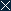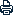Home | Sitemap | Contact | Chinese | CAS
 Search:
 About AMSS Research People International Cooperation News Societies & Journals Papers Resources Education & Training Join Us LinksLocation: Home >  Research >  Colloquia & Seminars
 （2019.3.5）Prof. Nicolas Burq：Almost sure global existence and scattering for the one dimensional Schrodinger equation (I)(II) (III)
 Author：
 ArticleSource：
 Update time： 2019-03-04CloseA A APrint

 Academy of Mathematics and Systems Science, CAS Colloquia & Seminars
 Speaker: Prof. Nicolas Burq，Université Paris-Sud , France Inviter: Title: Almost sure global existence and scattering for the one dimensional Schrodinger equation (I)(II) (III) Time & Venue: 2019.3.5 14:40-16:40；2019.3.8,10 15:00-17:00 N913 Abstract: In this mini course, I will give an introduction to the theory of random data nonlinear PDE’s, on one of the most simple example of dispersive PDE’s: the one dimensional nonlinear Schrodinger equation on the line $\mathbb{R}$. More precisely, I will define essentially on $L^2 (\mathbb {R})$, the space of initial data, probability measures for which I can describe the (nontrivial) evolution by the linear flow of the Schrodinger equation $$(i\partial_t+\partial _x2)u=0, (t,x) \in\mathbb{R} \times \mathbb{R}$$ These mesures are essentially supported on $L^2( \mathbb{R})$. Then I will show that the nonlinear equation $$(i\partial_t + \partial_x^2 ) u - |u|^{p-1} u =0, (t, x) \in \mathbb{R}\times \mathbb{R}$$ ，Is locally well posed on the support of the measure. Finally I will describe precisely the evolution by the nonlinear flow of the measure defined previously in terms of the linear evolution (quasi-invariance). Lastly I wil show how this description gives 1) (Almost sure) Global well posedness for p>1 and asymptotic behaviour of solutions (nonscattering type) 2) (Almost sure) scattering for p>3.” This is based on joint works with L. Thomann and N. Tzvetkov, and more recently with L. Thomann. The prerequisite in probability for the course are essentially elementary probability theory.
 Copyright@2008, All Rights Reserved, Academy of Mathematics and Systems Science, CAS Tel: 86-10-82541777 Fax: 86-10-82541972 E-mail: contact@amss.ac.cn